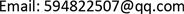1. 引言

2. 系统模型

Each symbol represents meanin

N网络中传感节点的个数
V网络中节点的总个数
r传输距离
m子网内节点个数
M调度方案包含的节点个数
(i, j)节点i和j之间的链路
ω采样周期
τ时隙大小
T调度模式包含的时隙数
C缓存队列大小
v节点发送速率
Rsink节点发送速率
S节点的调度模式
S调度方案
f数据流
si节点i发送数据包时间
tisink节点接收节点i发送的数据包时间

3. 问题陈述

min ∑ i = 1 N ( t i − s i ) (1)

∑ i = 1 N f i , j = 1       ∑ i = 1 N f j , i = 0 (2)

∑ i = 1 V f i , sin k = 1       ∑ i = 1 V f sin k , i = 0 (3)

m ≤ M (4)

R ≥ N v (5)

C ≥ N (6)

ω ≥ τ T N (7)

4. 基于优先级队列的免同步调度算法4.1. 分布式图染色

4.2. 优先级队列调度

5. 结果评估

6. 结论